## 6.4 Drag Force and Terminal Speed

### Learning Objectives

By the end of the section, you will be able to:

• Express the drag force mathematically
• Describe applications of the drag force
• Define terminal velocity
• Determine an object’s terminal velocity given its mass

Another interesting force in everyday life is the force of drag on an object when it is moving in a fluid (either a gas or a liquid). You feel the drag force when you move your hand through water. You might also feel it if you move your hand during a strong wind. The faster you move your hand, the harder it is to move. You feel a smaller drag force when you tilt your hand so only the side goes through the air—you have decreased the area of your hand that faces the direction of motion.

### Drag Forces

Like friction, the drag force always opposes the motion of an object. Unlike simple friction, the drag force is proportional to some function of the velocity of the object in that fluid. This functionality is complicated and depends upon the shape of the object, its size, its velocity, and the fluid it is in. For most large objects such as cyclists, cars, and baseballs not moving too slowly, the magnitude of the drag force ${F}_{\text{D}}$ is proportional to the square of the speed of the object. We can write this relationship mathematically as ${F}_{\text{D}}\propto {v}^{2}.$ When taking into account other factors, this relationship becomes

${F}_{\text{D}}=\frac{1}{2}C\rho A{v}^{2},$

where C is the drag coefficient, A is the area of the object facing the fluid, and $\rho$ is the density of the fluid. (Recall that density is mass per unit volume.) This equation can also be written in a more generalized fashion as ${F}_{\text{D}}=b{v}^{2},$ where b is a constant equivalent to $0.5C\rho A.$ We have set the exponent n for these equations as 2 because when an object is moving at high velocity through air, the magnitude of the drag force is proportional to the square of the speed. As we shall see in Fluid Mechanics, for small particles moving at low speeds in a fluid, the exponent n is equal to 1.

#### Drag Force

Drag force ${F}_{\text{D}}$ is proportional to the square of the speed of the object. Mathematically,

${F}_{\text{D}}=\frac{1}{2}C\,\rho \,A{v}^{2},$

where C is the drag coefficient, A is the area of the object facing the fluid, and $\rho$ is the density of the fluid.

Athletes as well as car designers seek to reduce the drag force to lower their race times ((Figure)). Aerodynamic shaping of an automobile can reduce the drag force and thus increase a car’s gas mileage.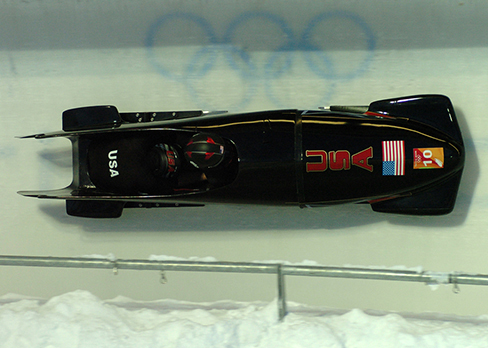Figure 6.29 From racing cars to bobsled racers, aerodynamic shaping is crucial to achieving top speeds. Bobsleds are designed for speed and are shaped like a bullet with tapered fins. (credit: “U.S. Army”/Wikimedia Commons)

The value of the drag coefficient C is determined empirically, usually with the use of a wind tunnel ((Figure)).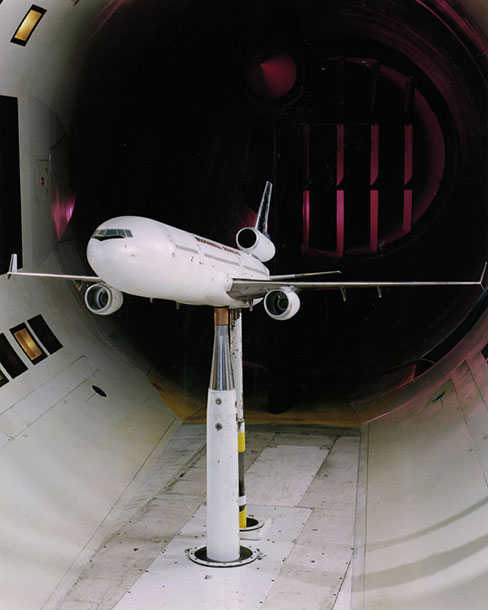Figure 6.30 NASA researchers test a model plane in a wind tunnel. (credit: NASA/Ames)

The drag coefficient can depend upon velocity, but we assume that it is a constant here. (Figure) lists some typical drag coefficients for a variety of objects. Notice that the drag coefficient is a dimensionless quantity. At highway speeds, over $50%$ of the power of a car is used to overcome air drag. The most fuel-efficient cruising speed is about 70–80 km/h (about 45–50 mi/h). For this reason, during the 1970s oil crisis in the United States, maximum speeds on highways were set at about 90 km/h (55 mi/h).

Typical Values of Drag Coefficient C
Object C
Airfoil 0.05
Toyota Camry 0.28
Ford Focus 0.32
Honda Civic 0.36
Ferrari Testarossa 0.37
Dodge Ram Pickup 0.43
Sphere 0.45
Hummer H2 SUV 0.64
Skydiver (feet first) 0.70
Bicycle 0.90
Skydiver (horizontal) 1.0
Circular flat plate 1.12

Substantial research is under way in the sporting world to minimize drag. The dimples on golf balls are being redesigned, as are the clothes that athletes wear. Bicycle racers and some swimmers and runners wear full bodysuits. Australian Cathy Freeman wore a full body suit in the 2000 Sydney Olympics and won a gold medal in the 400-m race. Many swimmers in the 2008 Beijing Olympics wore (Speedo) body suits; it might have made a difference in breaking many world records ((Figure)). Most elite swimmers (and cyclists) shave their body hair. Such innovations can have the effect of slicing away milliseconds in a race, sometimes making the difference between a gold and a silver medal. One consequence is that careful and precise guidelines must be continuously developed to maintain the integrity of the sport.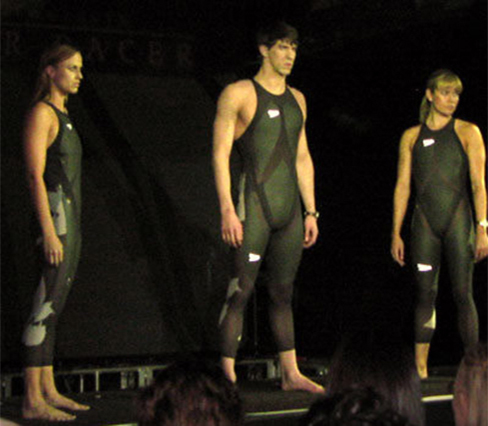Figure 6.31 Body suits, such as this LZR Racer Suit, have been credited with aiding in many world records after their release in 2008. Smoother “skin” and more compression forces on a swimmer’s body provide at least $10%$ less drag. (credit: NASA/Kathy Barnstorff)

### Terminal Velocity

Some interesting situations connected to Newton’s second law occur when considering the effects of drag forces upon a moving object. For instance, consider a skydiver falling through air under the influence of gravity. The two forces acting on him are the force of gravity and the drag force (ignoring the small buoyant force). The downward force of gravity remains constant regardless of the velocity at which the person is moving. However, as the person’s velocity increases, the magnitude of the drag force increases until the magnitude of the drag force is equal to the gravitational force, thus producing a net force of zero. A zero net force means that there is no acceleration, as shown by Newton’s second law. At this point, the person’s velocity remains constant and we say that the person has reached his terminal velocity $({v}_{\text{T}}).$ Since ${F}_{\text{D}}$ is proportional to the speed squared, a heavier skydiver must go faster for ${F}_{\text{D}}$ to equal his weight. Let’s see how this works out more quantitatively.

At the terminal velocity,

${F}_{\text{net}}=mg-{F}_{\text{D}}=ma=0.$

Thus,

$mg={F}_{\text{D}}.$

Using the equation for drag force, we have

$mg=\frac{1}{2}C\rho A{v}_{\text{T}}^{2}.$

Solving for the velocity, we obtain

${v}_{\text{T}}=\sqrt{\frac{2mg}{\rho CA}}.$

Assume the density of air is $\rho =1.21\,{\text{kg/m}}^{3}.$ A 75-kg skydiver descending head first has a cross-sectional area of approximately $A=0.18\,{\text{m}}^{2}$ and a drag coefficient of approximately $C=0.70$. We find that

${v}_{\text{T}}=\sqrt{\frac{2(75\,\text{kg})(9.80\,{\text{m/s}}^{2})}{(1.21\,{\text{kg/m}}^{3})(0.70)(0.18\,{\text{m}}^{2})}}=98\,\text{m/s}=350\,\text{km/h}\text{.}$

This means a skydiver with a mass of 75 kg achieves a terminal velocity of about 350 km/h while traveling in a pike (head first) position, minimizing the area and his drag. In a spread-eagle position, that terminal velocity may decrease to about 200 km/h as the area increases. This terminal velocity becomes much smaller after the parachute opens.

### Example

#### Terminal Velocity of a Skydiver

Find the terminal velocity of an 85-kg skydiver falling in a spread-eagle position.

#### Strategy

At terminal velocity, ${F}_{\text{net}}=0.$ Thus, the drag force on the skydiver must equal the force of gravity (the person’s weight). Using the equation of drag force, we find $mg=\frac{1}{2}\rho CA{v}^{2}.$

#### Solution

The terminal velocity ${v}_{\text{T}}$ can be written as

${v}_{\text{T}}=\sqrt{\frac{2mg}{\rho CA}}=\sqrt{\frac{2(85\,\text{kg})(9.80\,{\text{m/s}}^{2})}{(1.21\,{\text{kg/m}}^{3})(1.0)(0.70\,{\text{m}}^{2})}}=44\,\text{m/s}\text{.}$

#### Significance

This result is consistent with the value for ${v}_{\text{T}}$ mentioned earlier. The 75-kg skydiver going feet first had a terminal velocity of ${v}_{\text{T}}=98\,\text{m/s}\text{.}$ He weighed less but had a smaller frontal area and so a smaller drag due to the air.

Find the terminal velocity of a 50-kg skydiver falling in spread-eagle fashion.

The size of the object that is falling through air presents another interesting application of air drag. If you fall from a 5-m-high branch of a tree, you will likely get hurt—possibly fracturing a bone. However, a small squirrel does this all the time, without getting hurt. You do not reach a terminal velocity in such a short distance, but the squirrel does.

The following interesting quote on animal size and terminal velocity is from a 1928 essay by a British biologist, J. B. S. Haldane, titled “On Being the Right Size.”

“To the mouse and any smaller animal, [gravity] presents practically no dangers. You can drop a mouse down a thousand-yard mine shaft; and, on arriving at the bottom, it gets a slight shock and walks away, provided that the ground is fairly soft. A rat is killed, a man is broken, and a horse splashes. For the resistance presented to movement by the air is proportional to the surface of the moving object. Divide an animal’s length, breadth, and height each by ten; its weight is reduced to a thousandth, but its surface only to a hundredth. So the resistance to falling in the case of the small animal is relatively ten times greater than the driving force.”

The above quadratic dependence of air drag upon velocity does not hold if the object is very small, is going very slow, or is in a denser medium than air. Then we find that the drag force is proportional just to the velocity. This relationship is given by Stokes’ law.

#### Stokes’ Law

For a spherical object falling in a medium, the drag force is

${F}_{\text{s}}=6\pi r\eta v,$

where r is the radius of the object, $\eta$ is the viscosity of the fluid, and v is the object’s velocity.

Good examples of Stokes’ law are provided by microorganisms, pollen, and dust particles. Because each of these objects is so small, we find that many of these objects travel unaided only at a constant (terminal) velocity. Terminal velocities for bacteria (size about $1\,\text{μm})$ can be about $2\,\text{μm/s}\text{.}$ To move at a greater speed, many bacteria swim using flagella (organelles shaped like little tails) that are powered by little motors embedded in the cell.

Sediment in a lake can move at a greater terminal velocity (about $5\,\text{μm/s}),$ so it can take days for it to reach the bottom of the lake after being deposited on the surface.

If we compare animals living on land with those in water, you can see how drag has influenced evolution. Fish, dolphins, and even massive whales are streamlined in shape to reduce drag forces. Birds are streamlined and migratory species that fly large distances often have particular features such as long necks. Flocks of birds fly in the shape of a spearhead as the flock forms a streamlined pattern ((Figure)). In humans, one important example of streamlining is the shape of sperm, which need to be efficient in their use of energy.Figure 6.32 Geese fly in a V formation during their long migratory travels. This shape reduces drag and energy consumption for individual birds, and also allows them a better way to communicate. (credit: “Julo”/Wikimedia Commons)

In lecture demonstrations, we do measurements of the drag force on different objects. The objects are placed in a uniform airstream created by a fan. Calculate the Reynolds number and the drag coefficient.

### The Calculus of Velocity-Dependent Frictional Forces

When a body slides across a surface, the frictional force on it is approximately constant and given by ${\mu }_{\text{k}}N.$ Unfortunately, the frictional force on a body moving through a liquid or a gas does not behave so simply. This drag force is generally a complicated function of the body’s velocity. However, for a body moving in a straight line at moderate speeds through a liquid such as water, the frictional force can often be approximated by

${f}_{R}=\text{−}bv,$

where b is a constant whose value depends on the dimensions and shape of the body and the properties of the liquid, and v is the velocity of the body. Two situations for which the frictional force can be represented this equation are a motorboat moving through water and a small object falling slowly through a liquid.

Let’s consider the object falling through a liquid. The free-body diagram of this object with the positive direction downward is shown in (Figure). Newton’s second law in the vertical direction gives the differential equation

$mg-bv=m\frac{dv}{dt},$

where we have written the acceleration as $dv\text{/}dt.$ As v increases, the frictional force –bv increases until it matches mg. At this point, there is no acceleration and the velocity remains constant at the terminal velocity ${v}_{\text{T}}.$ From the previous equation,

$mg-b{v}_{\text{T}}=0,$

so

${v}_{\text{T}}=\frac{mg}{b}.$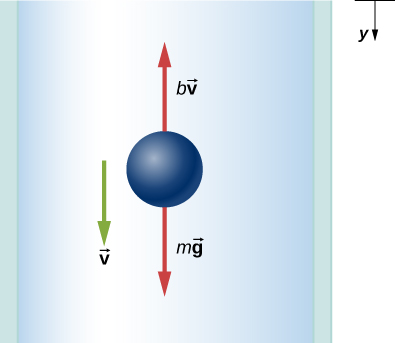Figure 6.33 Free-body diagram of an object falling through a resistive medium.

We can find the object’s velocity by integrating the differential equation for v. First, we rearrange terms in this equation to obtain

$\frac{dv}{g-(b\text{/}m)v}=dt.$

Assuming that $v=0\,\text{at}\,t=0,$ integration of this equation yields

${\int }_{0}^{v}\frac{d{v}^{\prime }}{g-(b\text{/}m){v}^{\prime }}={\int }_{0}^{t}d{t}^{\prime },$

or

${-\frac{m}{b}\text{ln}(g-\frac{b}{m}{v}^{\prime })|}_{0}^{v}={{t}^{\prime }|}_{0}^{t},$

where $v\text{‘}\,\text{and}\,t\text{‘}$ are dummy variables of integration. With the limits given, we find

$-\frac{m}{b}[\text{ln}(g-\frac{b}{m}v)-\text{ln}g]=t.$

Since $\text{ln}A-\text{ln}B=\text{ln}(A\text{/}B),$ and $\text{ln}(A\text{/}B)=x\,\text{implies}\,{e}^{x}=A\text{/}B,$ we obtain

$\frac{g-(bv\text{/}m)}{g}={e}^{\text{−}bt\text{/}m},$

and

$v=\frac{mg}{b}(1-{e}^{\text{−}bt\text{/}m}).$

Notice that as $t\to \infty ,v\to mg\text{/}b={v}_{\text{T}},$ which is the terminal velocity.

The position at any time may be found by integrating the equation for v. With $v=dy\text{/}dt,$

$dy=\frac{mg}{b}(1-{e}^{\text{−}bt\text{/}m})dt.$

Assuming $y=0\,\text{when}\,t=0,$

${\int }_{0}^{y}d{y}^{\prime }=\frac{mg}{b}{\int }_{0}^{t}(1-{e}^{\text{−}bt\text{‘}\text{/}m})d{t}^{\prime },$

which integrates to

$y=\frac{mg}{b}t+\frac{{m}^{2}g}{{b}^{2}}({e}^{\text{−}bt\text{/}m}-1).$

### Example

#### Effect of the Resistive Force on a Motorboat

A motorboat is moving across a lake at a speed ${v}_{0}$ when its motor suddenly freezes up and stops. The boat then slows down under the frictional force ${f}_{R}=\text{−}bv.$ (a) What are the velocity and position of the boat as functions of time? (b) If the boat slows down from 4.0 to 1.0 m/s in 10 s, how far does it travel before stopping?

#### Solution

1. With the motor stopped, the only horizontal force on the boat is ${f}_{R}=\text{−}bv,$ so from Newton’s second law,
$m\frac{dv}{dt}=\text{−}bv,$

which we can write as

$\frac{dv}{v}=-\frac{b}{m}dt.$

Integrating this equation between the time zero when the velocity is ${v}_{0}$ and the time t when the velocity is $v$, we have

${\int }_{0}^{v}\frac{d{v}^{\prime }}{{v}^{\prime }}=-\frac{b}{m}{\int }_{0}^{t}d{t}^{\prime }.$

Thus,

$\text{ln}\frac{v}{{v}_{0}}=-\frac{b}{m}t,$

which, since $\text{ln}A=x\,\text{implies}\,{e}^{x}=A,$ we can write this as

$v={v}_{0}{e}^{\text{−}bt\text{/}m}.$

Now from the definition of velocity,

$\frac{dx}{dt}={v}_{0}{e}^{\text{−}bt\text{/}m},$

so we have

$dx={v}_{0}{e}^{\text{−}bt\text{/}m}dt.$

With the initial position zero, we have

${\int }_{0}^{x}dx\text{‘}={v}_{0}{\int }_{0}^{t}{e}^{\text{−}bt\text{‘}\text{/}m}dt\text{‘},$

and

${x=-\frac{m{v}_{0}}{b}{e}^{\text{−}bt\text{‘}\text{/}m}|}_{0}^{t}=\frac{m{v}_{0}}{b}(1-{e}^{\text{−}bt\text{/}m}).$

As time increases, ${e}^{\text{−}bt\text{/}m}\to 0,$ and the position of the boat approaches a limiting value

${x}_{\text{max}}=\frac{m{v}_{0}}{b}.$

Although this tells us that the boat takes an infinite amount of time to reach ${x}_{\text{max}},$ the boat effectively stops after a reasonable time. For example, at $t=10m\text{/}b,$ we have

$v={v}_{0}{e}^{-10}\simeq 4.5\,×\,{10}^{-5}{v}_{0},$

whereas we also have

$x={x}_{\text{max}}(1-{e}^{-10})\simeq 0.99995{x}_{\text{max}}.$

Therefore, the boat’s velocity and position have essentially reached their final values.

2. With ${v}_{0}=4.0\,\text{m/s}$ and $v=1.0\,\text{m/s,}$ we have $1.0\,\text{m/s}=(4.0\,\text{m/s}){e}^{\text{−}(b\text{/}m)(10\,\text{s})},$ so
$\text{ln}\,0.25=\text{−}\text{ln}\,4.0=-\frac{b}{m}(10\,\text{s}),$

and

$\frac{b}{m}=\frac{1}{10}\text{ln}\,4.0\,{\text{s}}^{\text{-1}}=0.14\,{\text{s}}^{\text{-1}}\text{.}$

Now the boat’s limiting position is

${x}_{\text{max}}=\frac{m{v}_{0}}{b}=\frac{4.0\,\text{m/s}}{0.14\,{\text{s}}^{\text{−1}}}=29\,\text{m}\text{.}$

#### Significance

In the both of the previous examples, we found “limiting” values. The terminal velocity is the same as the limiting velocity, which is the velocity of the falling object after a (relatively) long time has passed. Similarly, the limiting distance of the boat is the distance the boat will travel after a long amount of time has passed. Due to the properties of exponential decay, the time involved to reach either of these values is actually not too long (certainly not an infinite amount of time!) but they are quickly found by taking the limit to infinity.

Suppose the resistive force of the air on a skydiver can be approximated by $f=\text{−}b{v}^{2}$. If the terminal velocity of a 100-kg skydiver is 60 m/s, what is the value of b?

### Summary

• Drag forces acting on an object moving in a fluid oppose the motion. For larger objects (such as a baseball) moving at a velocity in air, the drag force is determined using the drag coefficient (typical values are given in (Figure)), the area of the object facing the fluid, and the fluid density.
• For small objects (such as a bacterium) moving in a denser medium (such as water), the drag force is given by Stokes’ law.

### Key Equations

 Magnitude of static friction ${f}_{\text{s}}\le {\mu }_{\text{s}}N$ Magnitude of kinetic friction ${f}_{k}={\mu }_{k}N$ Centripetal force ${F}_{\text{c}}=m\frac{{v}^{2}}{r}\enspace\text{or}\enspace{F}_{\text{c}}=mr{\omega }^{2}$ Ideal angle of a banked curve $\text{tan}\,\theta =\frac{{v}^{2}}{rg}$ Drag force ${F}_{D}=\frac{1}{2}C\rho A{v}^{2}$ Stokes’ law ${F}_{\text{s}}=6\pi r\eta v$

### Conceptual Questions

Athletes such as swimmers and bicyclists wear body suits in competition. Formulate a list of pros and cons of such suits.

Two expressions were used for the drag force experienced by a moving object in a liquid. One depended upon the speed, while the other was proportional to the square of the speed. In which types of motion would each of these expressions be more applicable than the other one?

As cars travel, oil and gasoline leaks onto the road surface. If a light rain falls, what does this do to the control of the car? Does a heavy rain make any difference?

Why can a squirrel jump from a tree branch to the ground and run away undamaged, while a human could break a bone in such a fall?

### Problems

The terminal velocity of a person falling in air depends upon the weight and the area of the person facing the fluid. Find the terminal velocity (in meters per second and kilometers per hour) of an 80.0-kg skydiver falling in a pike (headfirst) position with a surface area of $0.140\,{\text{m}}^{2}$.

A 60.0-kg and a 90.0-kg skydiver jump from an airplane at an altitude of $6.00\,×\,{10}^{3}\text{m}$, both falling in the pike position. Make some assumption on their frontal areas and calculate their terminal velocities. How long will it take for each skydiver to reach the ground (assuming the time to reach terminal velocity is small)? Assume all values are accurate to three significant digits.

A 560-g squirrel with a surface area of $930\,{\text{cm}}^{2}$ falls from a 5.0-m tree to the ground. Estimate its terminal velocity. (Use a drag coefficient for a horizontal skydiver.) What will be the velocity of a 56-kg person hitting the ground, assuming no drag contribution in such a short distance?

To maintain a constant speed, the force provided by a car’s engine must equal the drag force plus the force of friction of the road (the rolling resistance). (a) What are the drag forces at 70 km/h and 100 km/h for a Toyota Camry? (Drag area is $0.70\,{\text{m}}^{2}$) (b) What is the drag force at 70 km/h and 100 km/h for a Hummer H2? (Drag area is $2.44\,{\text{m}}^{2})$ Assume all values are accurate to three significant digits.

By what factor does the drag force on a car increase as it goes from 65 to 110 km/h?

Calculate the velocity a spherical rain drop would achieve falling from 5.00 km (a) in the absence of air drag (b) with air drag. Take the size across of the drop to be 4 mm, the density to be $1.00\,×\,{10}^{3}\,{\text{kg/m}}^{3}$, and the surface area to be $\pi {r}^{2}$.

Using Stokes’ law, verify that the units for viscosity are kilograms per meter per second.

Find the terminal velocity of a spherical bacterium (diameter $2.00\,\text{μm}$) falling in water. You will first need to note that the drag force is equal to the weight at terminal velocity. Take the density of the bacterium to be $1.10\,×\,{10}^{3}\,{\text{kg/m}}^{3}$.

Stokes’ law describes sedimentation of particles in liquids and can be used to measure viscosity. Particles in liquids achieve terminal velocity quickly. One can measure the time it takes for a particle to fall a certain distance and then use Stokes’ law to calculate the viscosity of the liquid. Suppose a steel ball bearing (density $7.8\,×\,{10}^{3}\,{\text{kg/m}}^{3}$, diameter 3.0 mm) is dropped in a container of motor oil. It takes 12 s to fall a distance of 0.60 m. Calculate the viscosity of the oil.

Suppose that the resistive force of the air on a skydiver can be approximated by $f=\text{−}b{v}^{2}.$ If the terminal velocity of a 50.0-kg skydiver is 60.0 m/s, what is the value of b?

A small diamond of mass 10.0 g drops from a swimmer’s earring and falls through the water, reaching a terminal velocity of 2.0 m/s. (a) Assuming the frictional force on the diamond obeys $f=\text{−}bv,$ what is b? (b) How far does the diamond fall before it reaches 90 percent of its terminal speed?

(a) What is the final velocity of a car originally traveling at 50.0 km/h that decelerates at a rate of $0.400\,{\text{m/s}}^{2}$ for 50.0 s? Assume a coefficient of friction of 1.0. (b) What is unreasonable about the result? (c) Which premise is unreasonable, or which premises are inconsistent?

A 75.0-kg woman stands on a bathroom scale in an elevator that accelerates from rest to 30.0 m/s in 2.00 s. (a) Calculate the scale reading in newtons and compare it with her weight. (The scale exerts an upward force on her equal to its reading.) (b) What is unreasonable about the result? (c) Which premise is unreasonable, or which premises are inconsistent?

(a) Calculate the minimum coefficient of friction needed for a car to negotiate an unbanked 50.0 m radius curve at 30.0 m/s. (b) What is unreasonable about the result? (c) Which premises are unreasonable or inconsistent?

As shown below, if $M=5.50\,\text{kg,}$ what is the tension in string 1?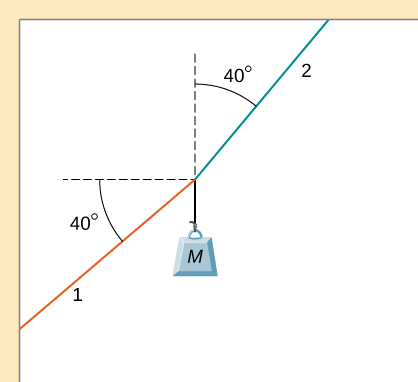As shown below, if $F=60.0\,\text{N}$ and $M=4.00\,\text{kg,}$ what is the magnitude of the acceleration of the suspended object? All surfaces are frictionless.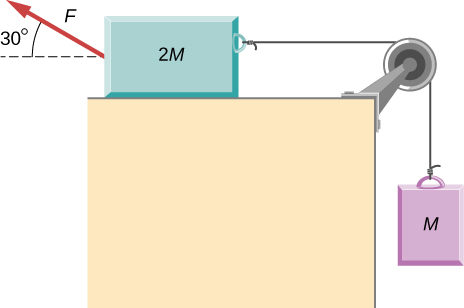As shown below, if $M=6.0\,\text{kg,}$ what is the tension in the connecting string? The pulley and all surfaces are frictionless.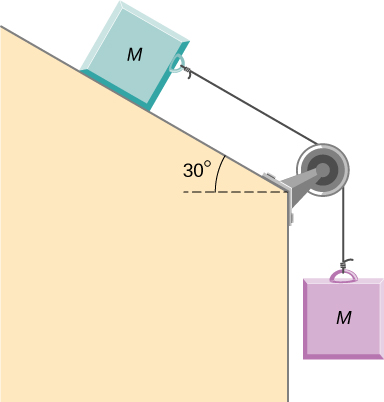A small space probe is released from a spaceship. The space probe has mass 20.0 kg and contains 90.0 kg of fuel. It starts from rest in deep space, from the origin of a coordinate system based on the spaceship, and burns fuel at the rate of 3.00 kg/s. The engine provides a constant thrust of 120.0 N. (a) Write an expression for the mass of the space probe as a function of time, between 0 and 30 seconds, assuming that the engine ignites fuel beginning at $t=0.$ (b) What is the velocity after 15.0 s? (c) What is the position of the space probe after 15.0 s, with initial position at the origin? (d) Write an expression for the position as a function of time, for $t>30.0\,\text{s}\text{.}$

A half-full recycling bin has mass 3.0 kg and is pushed up a $40.0\text{°}$ incline with constant speed under the action of a 26-N force acting up and parallel to the incline. The incline has friction. What magnitude force must act up and parallel to the incline for the bin to move down the incline at constant velocity?

A child has mass 6.0 kg and slides down a $35\text{°}$ incline with constant speed under the action of a 34-N force acting up and parallel to the incline. What is the coefficient of kinetic friction between the child and the surface of the incline?

The two barges shown here are coupled by a cable of negligible mass. The mass of the front barge is $2.00\,×\,{10}^{3}\,\text{kg}$ and the mass of the rear barge is $3.00\,×\,{10}^{3}\,\text{kg}\text{.}$ A tugboat pulls the front barge with a horizontal force of magnitude $20.0\,×\,{10}^{3}\,\text{N,}$ and the frictional forces of the water on the front and rear barges are $8.00\,×\,{10}^{3}\,\text{N}$ and $10.0\,×\,{10}^{3}\,\text{N,}$ respectively. Find the horizontal acceleration of the barges and the tension in the connecting cable.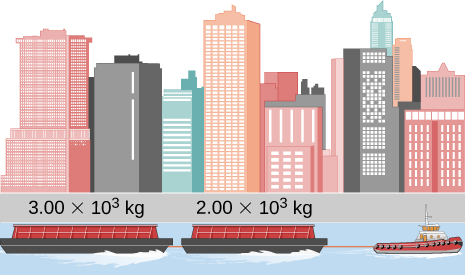If the order of the barges of the preceding exercise is reversed so that the tugboat pulls the $3.00\,×\,{10}^{3}\text{-kg}$ barge with a force of $20.0\,×\,{10}^{3}\,\text{N},$ what are the acceleration of the barges and the tension in the coupling cable?

An object with mass m moves along the x-axis. Its position at any time is given by $x(t)=p{t}^{3}+q{t}^{2}$ where p and q are constants. Find the net force on this object for any time t.

A helicopter with mass $2.35\,×\,{10}^{4}\,\text{kg}$ has a position given by $\overset{\to }{r}(t)=(0.020\,{t}^{3})\hat{i}+(2.2t)\hat{j}-(0.060\,{t}^{2})\hat{k}.$ Find the net force on the helicopter at $t=3.0\,\text{s}\text{.}$

Located at the origin, an electric car of mass m is at rest and in equilibrium. A time dependent force of $\overset{\to }{F}(t)$ is applied at time $t=0$, and its components are ${F}_{x}(t)=p+nt$ and ${F}_{y}(t)=qt$ where p, q, and n are constants. Find the position $\overset{\to }{r}(t)$ and velocity $\overset{\to }{v}(t)$ as functions of time t.

A particle of mass m is located at the origin. It is at rest and in equilibrium. A time-dependent force of $\overset{\to }{F}(t)$ is applied at time $t=0$, and its components are ${F}_{x}(t)=pt$ and ${F}_{y}(t)=n+qt$ where p, q, and n are constants. Find the position $\overset{\to }{r}(t)$ and velocity $\overset{\to }{v}(t)$ as functions of time t.

A 2.0-kg object has a velocity of $4.0\hat{i}\,\text{m/s}$ at $t=0.$ A constant resultant force of $(2.0\hat{i}+4.0\hat{j})\,\text{N}$ then acts on the object for 3.0 s. What is the magnitude of the object’s velocity at the end of the 3.0-s interval?

A 1.5-kg mass has an acceleration of $(4.0\hat{i}-3.0\hat{j})\,{\text{m/s}}^{2}.$ Only two forces act on the mass. If one of the forces is $(2.0\hat{i}-1.4\hat{j})\,\text{N,}$ what is the magnitude of the other force?

A box is dropped onto a conveyor belt moving at 3.4 m/s. If the coefficient of friction between the box and the belt is 0.27, how long will it take before the box moves without slipping?

Shown below is a 10.0-kg block being pushed by a horizontal force $\overset{\to }{F}$ of magnitude 200.0 N. The coefficient of kinetic friction between the two surfaces is 0.50. Find the acceleration of the block.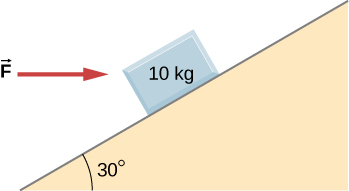As shown below, the mass of block 1 is ${m}_{1}=4.0\,\text{kg,}$ while the mass of block 2 is ${m}_{2}=8.0\,\text{kg}\text{.}$ The coefficient of friction between ${m}_{1}$ and the inclined surface is ${\mu }_{\text{k}}=0.40.$ What is the acceleration of the system?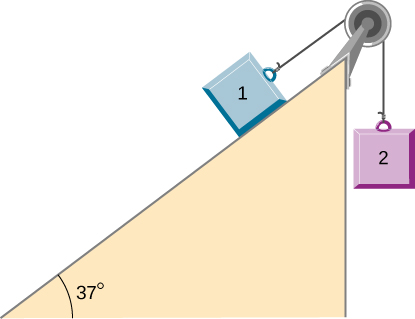A student is attempting to move a 30-kg mini-fridge into her dorm room. During a moment of inattention, the mini-fridge slides down a 35 degree incline at constant speed when she applies a force of 25 N acting up and parallel to the incline. What is the coefficient of kinetic friction between the fridge and the surface of the incline?

A crate of mass 100.0 kg rests on a rough surface inclined at an angle of $37.0\text{°}$ with the horizontal. A massless rope to which a force can be applied parallel to the surface is attached to the crate and leads to the top of the incline. In its present state, the crate is just ready to slip and start to move down the plane. The coefficient of friction is $80%$ of that for the static case. (a) What is the coefficient of static friction? (b) What is the maximum force that can be applied upward along the plane on the rope and not move the block? (c) With a slightly greater applied force, the block will slide up the plane. Once it begins to move, what is its acceleration and what reduced force is necessary to keep it moving upward at constant speed? (d) If the block is given a slight nudge to get it started down the plane, what will be its acceleration in that direction? (e) Once the block begins to slide downward, what upward force on the rope is required to keep the block from accelerating downward?

A car is moving at high speed along a highway when the driver makes an emergency braking. The wheels become locked (stop rolling), and the resulting skid marks are 32.0 meters long. If the coefficient of kinetic friction between tires and road is 0.550, and the acceleration was constant during braking, how fast was the car going when the wheels became locked?

A crate having mass 50.0 kg falls horizontally off the back of the flatbed truck, which is traveling at 100 km/h. Find the value of the coefficient of kinetic friction between the road and crate if the crate slides 50 m on the road in coming to rest. The initial speed of the crate is the same as the truck, 100 km/h.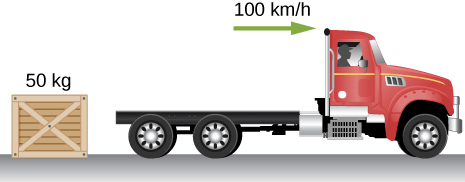A 15-kg sled is pulled across a horizontal, snow-covered surface by a force applied to a rope at 30 degrees with the horizontal. The coefficient of kinetic friction between the sled and the snow is 0.20. (a) If the force is 33 N, what is the horizontal acceleration of the sled? (b) What must the force be in order to pull the sled at constant velocity?

A 30.0-g ball at the end of a string is swung in a vertical circle with a radius of 25.0 cm. The rotational velocity is 200.0 cm/s. Find the tension in the string: (a) at the top of the circle, (b) at the bottom of the circle, and (c) at a distance of 12.5 cm from the center of the circle $(r=12.5\,\text{cm}).$

A particle of mass 0.50 kg starts moves through a circular path in the xy-plane with a position given by $\overset{\to }{r}(t)=(4.0\,\text{cos}\,3t)\hat{i}+(4.0\,\text{sin}\,3t)\hat{j}$ where r is in meters and t is in seconds. (a) Find the velocity and acceleration vectors as functions of time. (b) Show that the acceleration vector always points toward the center of the circle (and thus represents centripetal acceleration). (c) Find the centripetal force vector as a function of time.

A stunt cyclist rides on the interior of a cylinder 12 m in radius. The coefficient of static friction between the tires and the wall is 0.68. Find the value of the minimum speed for the cyclist to perform the stunt.

When a body of mass 0.25 kg is attached to a vertical massless spring, it is extended 5.0 cm from its unstretched length of 4.0 cm. The body and spring are placed on a horizontal frictionless surface and rotated about the held end of the spring at 2.0 rev/s. How far is the spring stretched?

Railroad tracks follow a circular curve of radius 500.0 m and are banked at an angle of $5.00\text{°}$. For trains of what speed are these tracks designed?

A plumb bob hangs from the roof of a railroad car. The car rounds a circular track of radius 300.0 m at a speed of 90.0 km/h. At what angle relative to the vertical does the plumb bob hang?

An airplane flies at 120.0 m/s and banks at a $30\text{°}$ angle. If its mass is $2.50\,×\,{10}^{3}\,\text{kg,}$ (a) what is the magnitude of the lift force? (b) what is the radius of the turn?

The position of a particle is given by $\overset{\to }{r}(t)=A(\text{cos}\,\omega t\hat{i}+\text{sin}\,\omega t\hat{j}),$ where $\omega$ is a constant. (a) Show that the particle moves in a circle of radius A. (b) Calculate $d\overset{\to }{r}\text{/}dt$ and then show that the speed of the particle is a constant ${A}_{\omega }.$ (c) Determine ${d}^{2}\overset{\to }{r}\text{/}d{t}^{2}$ and show that a is given by${a}_{\text{c}}=r{\omega }^{2}.$ (d) Calculate the centripetal force on the particle. [Hint: For (b) and (c), you will need to use $(d\text{/}dt)(\text{cos}\,\omega t)=\text{−}\omega \,\text{sin}\,\omega t$ and $(d\text{/}dt)(\text{sin}\,\omega t)=\omega \,\text{cos}\,\omega t.$

Two blocks connected by a string are pulled across a horizontal surface by a force applied to one of the blocks, as shown below. The coefficient of kinetic friction between the blocks and the surface is 0.25. If each block has an acceleration of $2.0\,{\text{m/s}}^{2}$ to the right, what is the magnitude F of the applied force?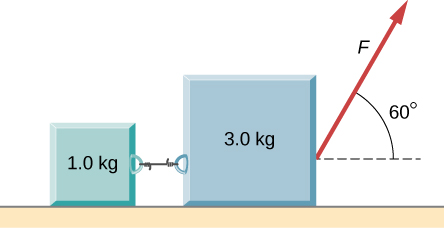As shown below, the coefficient of kinetic friction between the surface and the larger block is 0.20, and the coefficient of kinetic friction between the surface and the smaller block is 0.30. If $F=10\,\text{N}$ and $M=1.0\,\text{kg}$, what is the tension in the connecting string?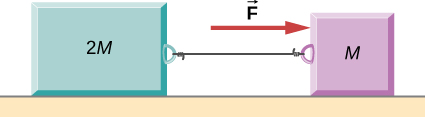In the figure, the coefficient of kinetic friction between the surface and the blocks is ${\mu }_{\text{k}}.$ If $M=1.0\,\text{kg,}$ find an expression for the magnitude of the acceleration of either block (in terms of F, ${\mu }_{\text{k}},$ and g).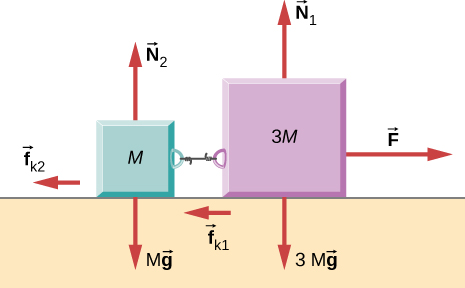Two blocks are stacked as shown below, and rest on a frictionless surface. There is friction between the two blocks (coefficient of friction $\mu$). An external force is applied to the top block at an angle $\theta$ with the horizontal. What is the maximum force F that can be applied for the two blocks to move together?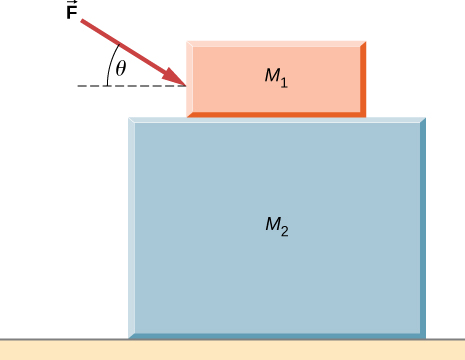A box rests on the (horizontal) back of a truck. The coefficient of static friction between the box and the surface on which it rests is 0.24. What maximum distance can the truck travel (starting from rest and moving horizontally with constant acceleration) in 3.0 s without having the box slide?

A double-incline plane is shown below. The coefficient of friction on the left surface is 0.30, and on the right surface 0.16. Calculate the acceleration of the system.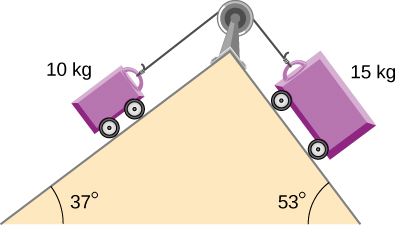### Challenge Problems

In a later chapter, you will find that the weight of a particle varies with altitude such that $w=\frac{mg{r}_{0}{}^{2}}{{r}^{2}}$ where ${r}_{0}{}^{}$ is the radius of Earth and r is the distance from Earth’s center. If the particle is fired vertically with velocity ${v}_{0}{}^{}$ from Earth’s surface, determine its velocity as a function of position r. (Hint: use ${a}^{}dr={v}^{}dv,$ the rearrangement mentioned in the text.)

A large centrifuge, like the one shown below, is used to expose aspiring astronauts to accelerations similar to those experienced in rocket launches and atmospheric reentries. (a) At what angular velocity is the centripetal acceleration 10g if the rider is 15.0 m from the center of rotation? (b) The rider’s cage hangs on a pivot at the end of the arm, allowing it to swing outward during rotation as shown in the bottom accompanying figure. At what angle $\theta$ below the horizontal will the cage hang when the centripetal acceleration is 10g? (Hint: The arm supplies centripetal force and supports the weight of the cage. Draw a free-body diagram of the forces to see what the angle $\theta$ should be.)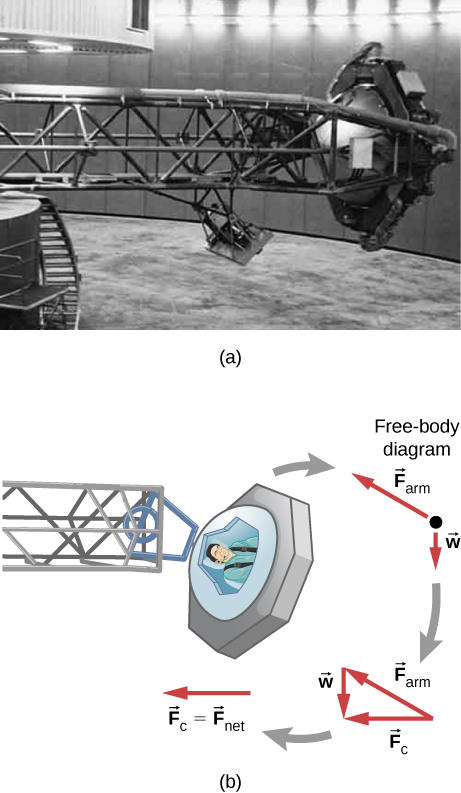A car of mass 1000.0 kg is traveling along a level road at 100.0 km/h when its brakes are applied. Calculate the stopping distance if the coefficient of kinetic friction of the tires is 0.500. Neglect air resistance. (Hint: since the distance traveled is of interest rather than the time, x is the desired independent variable and not t. Use the Chain Rule to change the variable: $\frac{dv}{dt}=\frac{dv}{dx}\,\frac{dx}{dt}=v\frac{dv}{dx}.)$

An airplane flying at 200.0 m/s makes a turn that takes 4.0 min. What bank angle is required? What is the percentage increase in the perceived weight of the passengers?

A skydiver is at an altitude of 1520 m. After 10.0 seconds of free fall, he opens his parachute and finds that the air resistance, ${F}_{\text{D}}$, is given by the formula ${F}_{\text{D}}=\text{−}bv,$ where b is a constant and v is the velocity. If $b=0.750,$ and the mass of the skydiver is 82.0 kg, first set up differential equations for the velocity and the position, and then find: (a) the speed of the skydiver when the parachute opens, (b) the distance fallen before the parachute opens, (c) the terminal velocity after the parachute opens (find the limiting velocity), and (d) the time the skydiver is in the air after the parachute opens.

In a television commercial, a small, spherical bead of mass 4.00 g is released from rest at $t=0$ in a bottle of liquid shampoo. The terminal speed is observed to be 2.00 cm/s. Find (a) the value of the constant b in the equation $v=\frac{mg}{b}(1-{e}^{\text{−}bt\text{/}m}),$ and (b) the value of the resistive force when the bead reaches terminal speed.

A boater and motor boat are at rest on a lake. Together, they have mass 200.0 kg. If the thrust of the motor is a constant force of 40.0 N in the direction of motion, and if the resistive force of the water is numerically equivalent to 2 times the speed v of the boat, set up and solve the differential equation to find: (a) the velocity of the boat at time t; (b) the limiting velocity (the velocity after a long time has passed).

### Glossary

drag force
force that always opposes the motion of an object in a fluid; unlike simple friction, the drag force is proportional to some function of the velocity of the object in that fluid
terminal velocity
constant velocity achieved by a falling object, which occurs when the weight of the object is balanced by the upward drag force